Courses
Courses for Kids
Free study material
Free LIVE classes
More

# Shapes and Designs Class 3 Notes CBSE Maths Chapter 5 (Free PDF Download)LIVE
Join Vedantu’s FREE Mastercalss

## NCERT Class 3 Maths Chapter 5 Shapes and Designs Revision Notes

One of the fascinating chapters of the CBSE Class 3 Maths syllabus, Shapes and Designs teaches students the concepts of edges, lines, folds, and shapes. They will also learn the different types of shapes and how to make designs with them in this chapter. To make it easier for the young students of Class 3, our experts have formulated Shape and Designs revision notes.

The easy language of these revision notes will enable students to comprehend these geometrical concepts effectively. Students will learn how to answer questions related to lines, edges, corners, shapes, positions, locations, etc of objects from these revision notes. The images of Shape and Designs are illustrated in these notes for a better understanding of students.

Also, check CBSE Class 3 Maths revision notes for other chapters:

Last updated date: 28th Sep 2023
Total views: 145.5k
Views today: 3.45k

## Revision Notes Class 3 Mathematics Chapter 5 - Shape and Designs

### Shapes

• Shapes provide an object's boundary.

• A boundary is created by mixing the curves, points, and line segments to form shapes.

• Examples of shapes: circle, square, rectangle, triangle, and so on.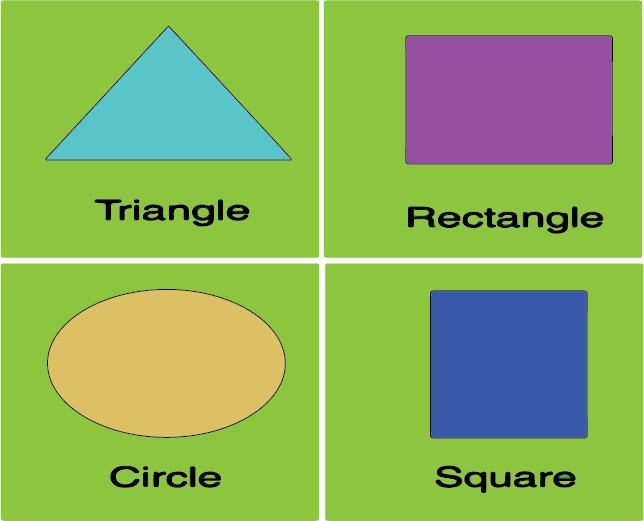Different shapes

### Different Types of Shapes

The following classification is used to determine the base of each shape:

• Open Shapes: Open shapes are made up of line segments or curves that do not intersect and are not continuous.

Example: An open shape is represented by the letter C.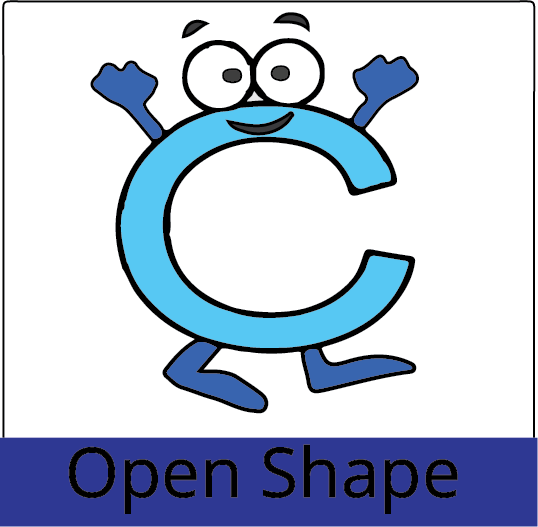Open shape

• Closed Shapes: Closed shapes are those that can be traced without breaking. They both begin and end in the same location.

Example: A closed shape is represented by the letter D.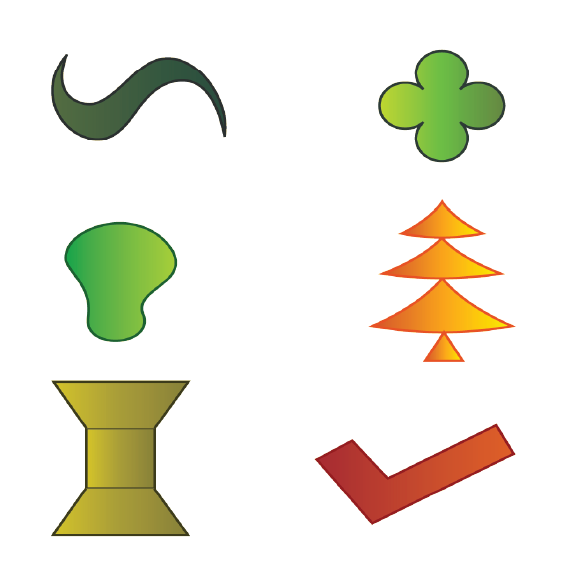Closed Shape

Solved Example 1: Is alphabet (A) a closed shape?

Ans: No, alphabet (A) is not a closed shape.

Solved Example 2: Give 3 examples of closed shape alphabets?

Ans: 3 examples of closed shape alphabets are (O), (B), and (D).

Solved Example 3: Give 2 examples of open shape alphabets?

Ans: 2 examples of open shape alphabets are (U), and (S).

### Circle

• A circle is defined as a closed-form.

• It's classified as a two-dimensional geometric shape with a round shape.

• There are no lines or corners on it.

• For example, a vehicle's steering wheel, a pizza base, or a dartboard.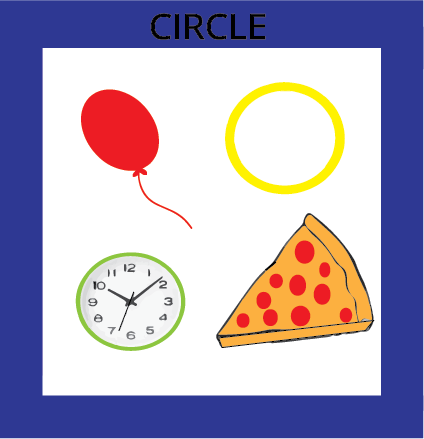Circle

### Oval

• An oval is a slightly extended form that resembles a circle.

• There are no straight lines or corners on it.

• The number zero, for example (0).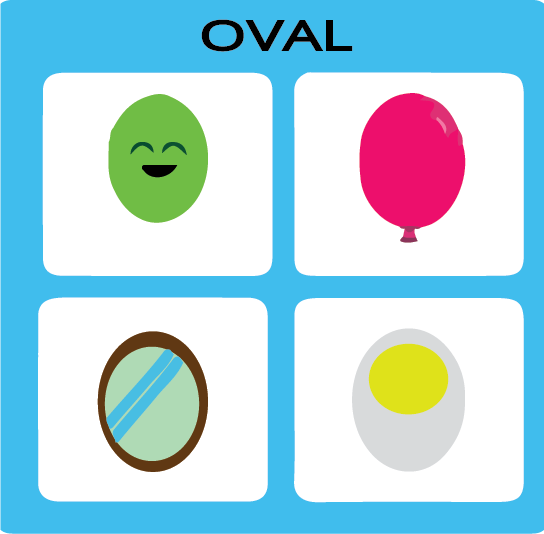Oval

### Square

• It is a closed two-dimensional shape with four sides.

• Each side is measured at the same length.

• A chessboard and a carrom board, for example.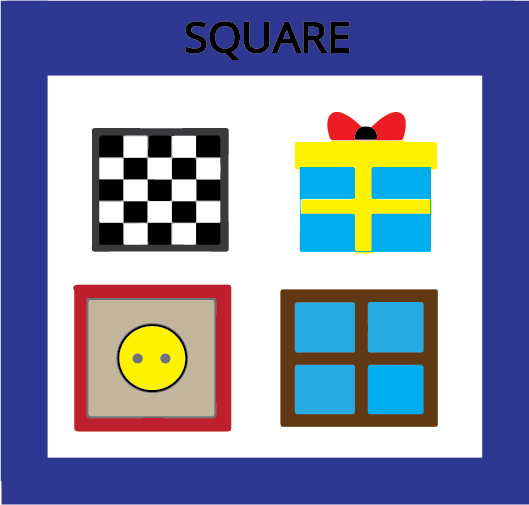Square

### Triangle

• A triangle is a three-sided geometric shape that is classified as two-dimensional.

• For example, one slice of cheese burst pizza in the shape of sour nachos.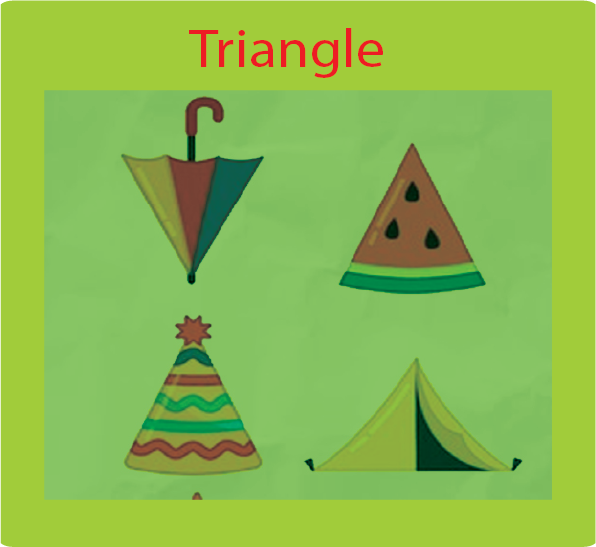Triangle

### Rectangle

• It is a shape with four sides.

• It's a two-dimensional geometric shape with opposite sides that are the same length.

• For example, a laptop screen, a touch screen phone, and so on.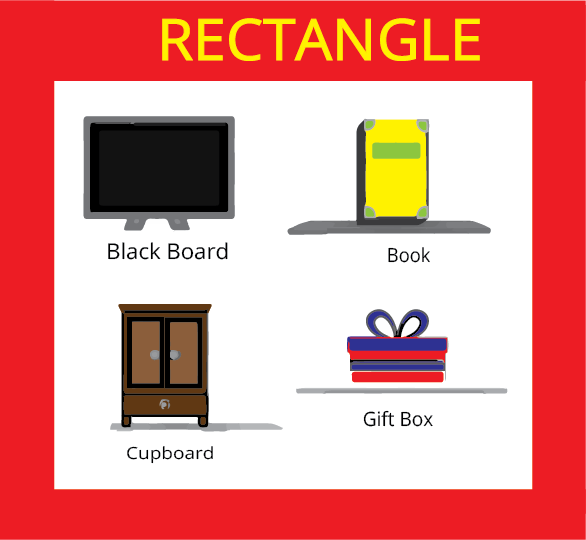Rectangle

### Counting of Triangles

• For counting triangles, add numbers to the base of smaller triangles. Then add the base numbers to calculate the total number of triangles.

Solved Example 1: Annie wants to count the number of triangles present in the given image?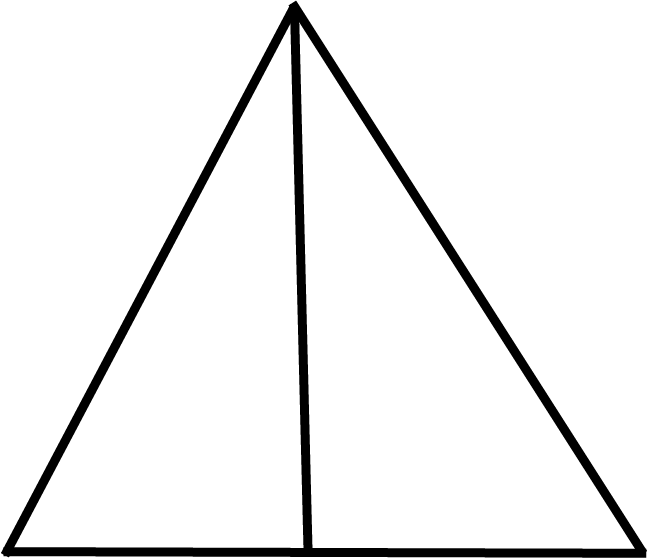Counting Rectangles

Ans: Here, Annie wants to count the total number of triangles present in the image. So, Annie will use the concept of counting shapes to find how many triangles are present:

• Step1: Identify the different shapes present in the image. Here, in the above image, only triangles are present.

• Step2: Add the numbers to the base of the small triangles.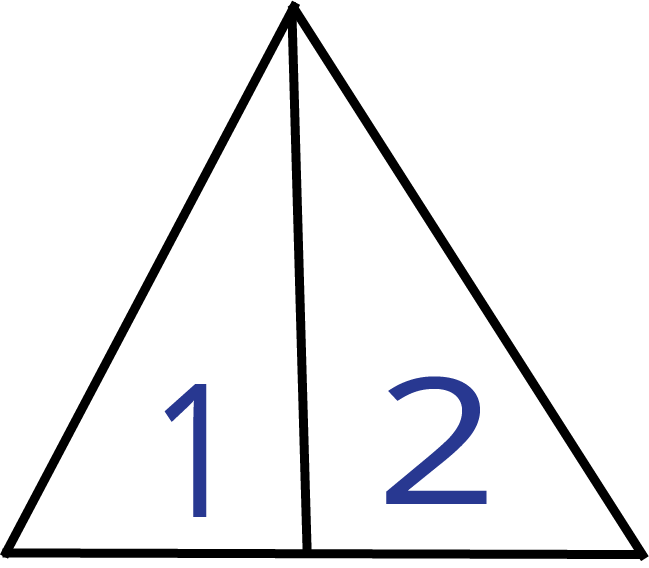Base Triangles

• Step3: To calculate the total number of triangles, add base numbers.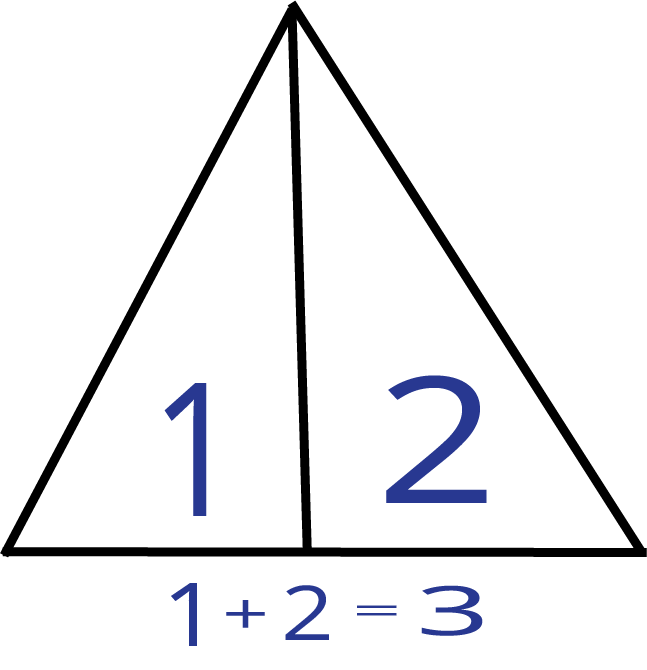Total Triangles

• Total number of triangles = 3

• So, the total number of triangles present in the image is 3.

Solved Example 2: Count the number of triangles present in the given image?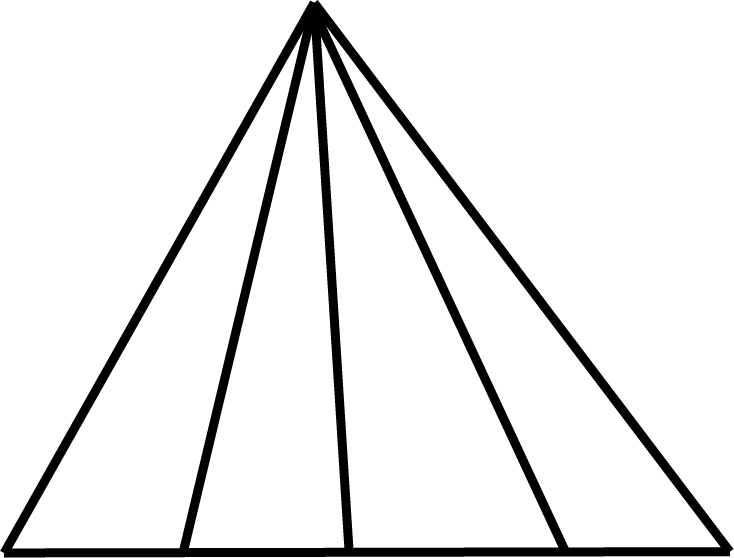Counting Triangles

Ans: Here, we want to count the total number of triangles present in the image. So, we will use the concept of counting shapes to find how many triangles are present:

• Step1: Identify the different shapes present in the image. Here, in the above image, only triangles are present.

• Step2: Add the numbers to the base of the small triangles.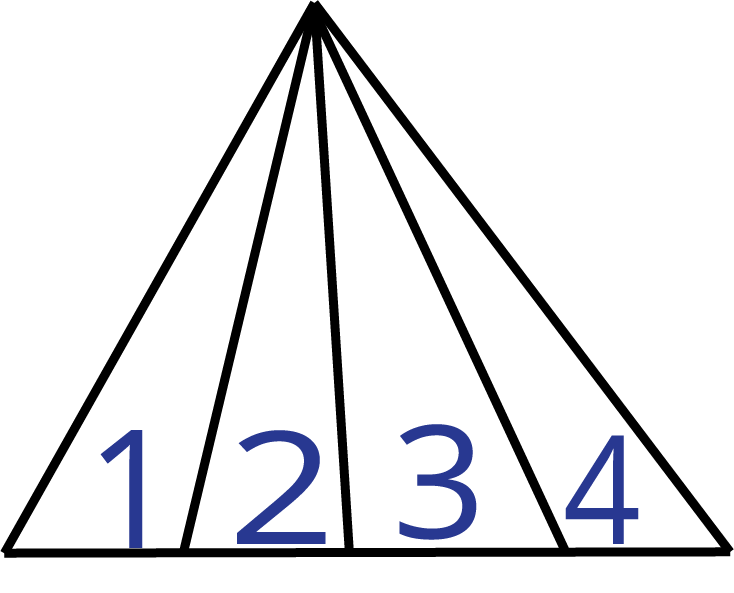Base Triangle

• Step3: To calculate the total number of triangles, add base numbers.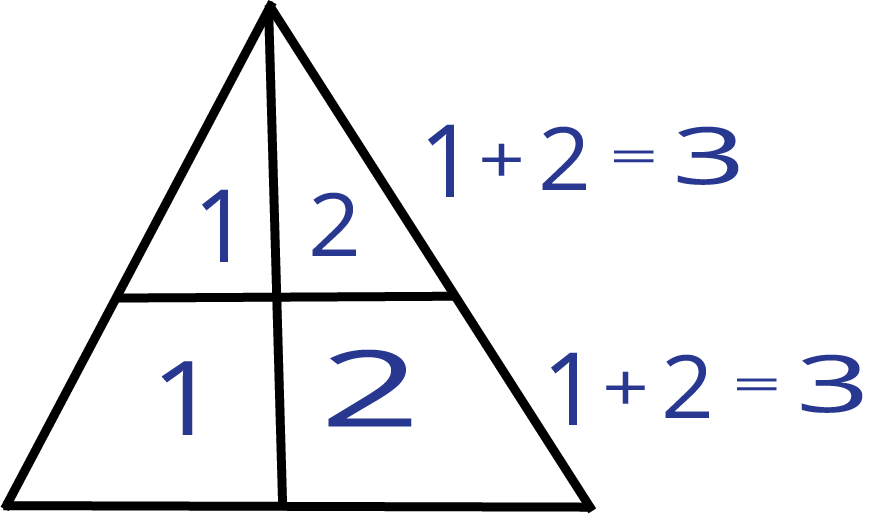Addition of base numbers of triangles

• Total number of triangles = 10

• So, the total number of triangles present in the image is 10.

Solved Example 3: Sam wants to count the number of triangles present in the given image?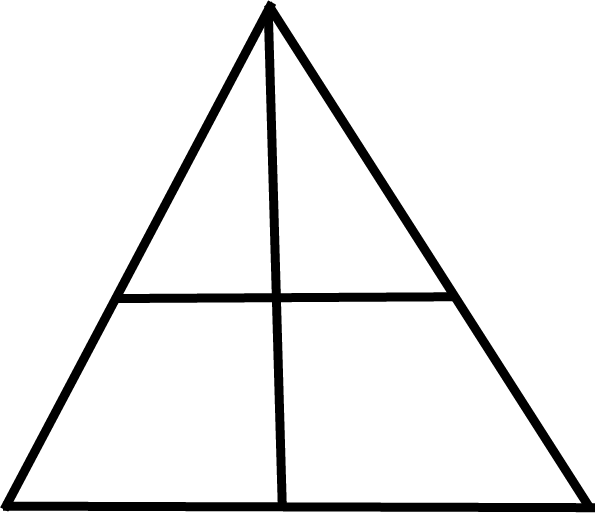Counting Triangle

Ans: Here, Sam wants to count the total number of triangles present in the image. So, Sam will use the concept of counting shapes to find how many triangles are present:

• Step1: Identify the different shapes present in the image. Here, in the above image, only triangles are present.

• Step2: Add the numbers to the base of the small triangles. Here, we have 2 small triangles in the first layer and 2 small triangles in the second layer.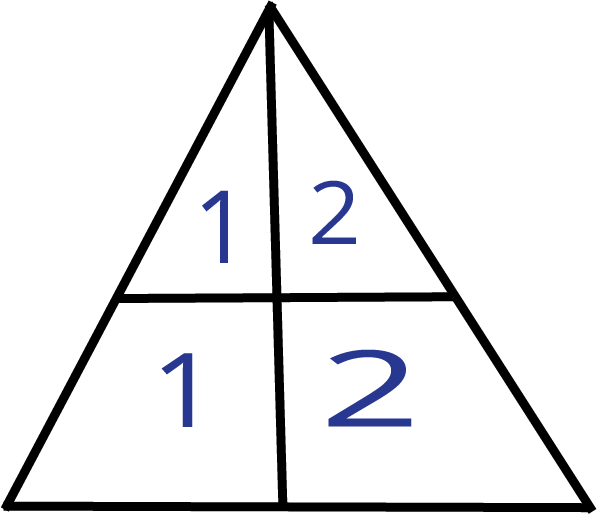Base Triangle

• Step3: To calculate the total number of triangles, add base numbers present on each layer.• Number of triangles in the first layer  = 3

• Number of triangles in the second layer = 3

• Total number of triangles in both layers = 3+3 = 6

• So, the total number of triangles present in the image is 6.

### Edges of Shapes

• They are the lines that outline a shape.

• For example, a square has 4 edges.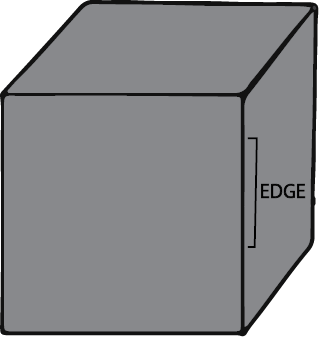Edges of a square

### Corners of Shapes

• They are the pointed parts or vertices where edges meet.

• For example, a rectangle has 4 corners.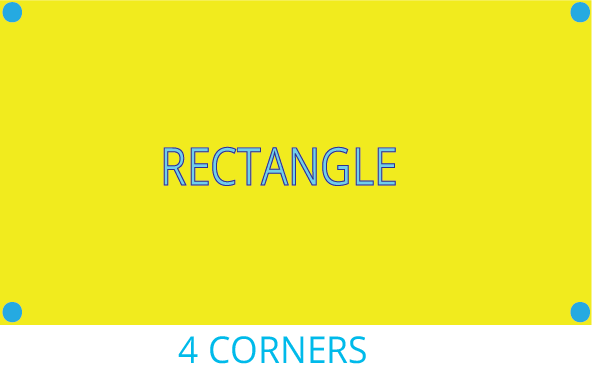Corners of a rectangle

Solved Example 1: How many edges does a triangle have?

Ans: A triangle has 3 edges.

Solved Example 2: How many edges does a ball have?

Ans: A ball has zero edges.

Solved Example 3: How many corners does a square have?

Ans: A square has 4 corners.

Solved Example 4: How many corners does an oval have?

Ans: An oval has 0 corners.

### Patterns

• We may make a variety of patterns or designs with different basic shapes.

• Weaving patterns, tiling patterns, and floor patterns are all examples of designs.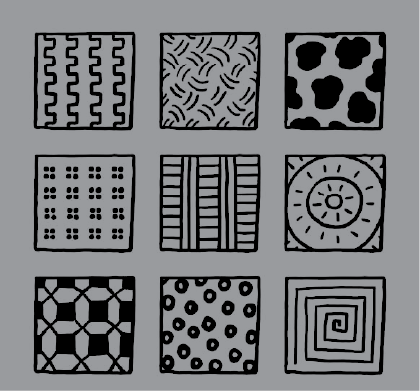Different patterns

### Practice Questions

Q1. Write (A) for closed shape and (B) for open shape in the following image.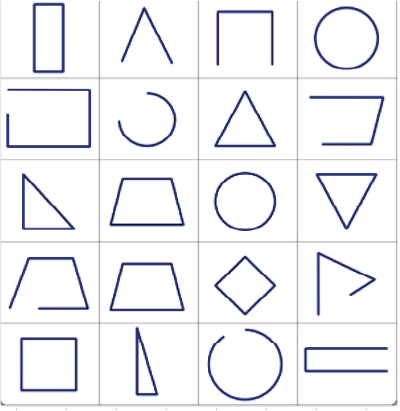Open and closed shapes

Q2. Count the number of different basic shapes in the following image.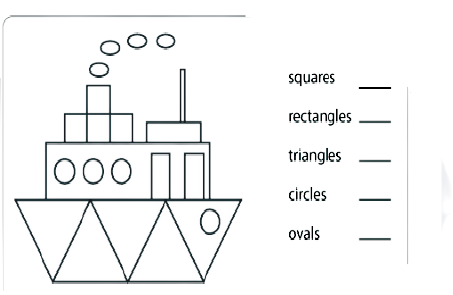Different basic shapes

Ans 1.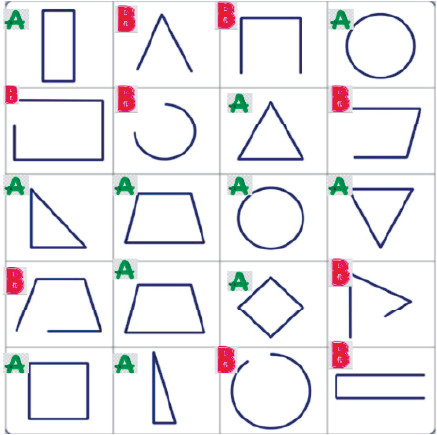Open and closed shapes

Ans 2.

• Number of squares: 4

• Number of rectangles: 5

• Number of triangles: 5

• Number of circles: 4

• Number of ovals: 4

## Importance of Shape and Design Class 3 Chapter 5

• This chapter has been formulated by the NCERT to introduce various geometrical concepts to Class 3 students. By studying this chapter, students will become ready for the advanced geometric concepts taught in the higher classes.

• Students will be able to identify and differentiate between lines, edges, corners, positions, locations, etc.

• The image illustration will enable students to imbibe geometrical concepts of straight lines, curved lines, edges, corners, etc.

• There are excellent activities covered in this chapter that enables students to realize how folds and creases on paper can create new shapes. They will learn how to create new corners, lines, edges, curves, angles, etc., and will gain hands-on geometrical skills.

• They will also solve various common puzzles to understand the edges and corners of shapes. They will also become skilled in making new objects using given shapes.

• On moving further, students will get to know what patterns are and how they can be recognized.

## Benefits of Class 3 Maths Chapter 5 Shapes and Designs Revision Notes

By using these revision notes for Class 3 Maths Chapter 5, students will be benefitted in the following ways.

• Understanding different types of shapes and their differences

• Learning how to draw or make shapes on or with paper respectively

• A simple explanation of the concepts will help you to make a maths border design.

• You can easily complete the syllabus for Class 3 drawing for this chapter at home and prepare for the exams.

• The chapter will also help students to realize geometric shapes according to the number of sides and symmetry.

By using the revision notes, you can also find the answer to what name is given for a shape with 10 sides. Solving these questions will enhance the intellect and comprehension skills of a Class 3 student.

## Conclusion

The Class 3 CBSE Maths Chapter 5 - "Shapes and Designs" notes, available as a free PDF download, are a valuable educational resource for both students and educators. These notes provide a comprehensive overview of geometric concepts, making learning engaging and accessible for young learners. With clear explanations, visual aids, and interactive examples, students can grasp the fundamentals of shapes and designs with ease. These free PDF notes empower both teachers and parents to facilitate effective learning experiences. They not only promote mathematical proficiency but also encourage creativity and problem-solving skills. In essence, the "Shapes and Designs" Class 3 CBSE Maths notes serve as a vital tool for nurturing a strong mathematical foundation in students while fostering a love for mathematics.

## FAQs on Shapes and Designs Class 3 Notes CBSE Maths Chapter 5 (Free PDF Download)

1.  How can I recognize the different edges of a shape?

Refer to the revision notes on Vedantu for NCERT Class 3 Maths Chapter 5 and understand the meaning of sides first. Learn to recognize the edges of a given shape and find its geometrical significance.

2.  How can I prepare for Chapter 5 Shapes and Designs before an exam?

Refer to the revision notes formulated by the experts for quick recall of concepts. Go through these notes before an exam and remember how to solve the problems.

The revision notes are the precise presentation of the basic geometrical concepts by sticking to the CBSE standards for Class 3. It is a good study material you can use to resolve queries.

4. Are there any interactive elements or exercises in the PDF of Shapes and Designs Class 3 Notes?

It depends on the source of the PDF notes. Some PDFs may include interactive elements and exercises, while others may provide more of a conceptual overview. It's essential to review the content before using it to ensure it meets your specific learning needs.

5. Can I use Shapes and Designs Class 3 Notes to supplement my child's learning at home?

Absolutely. These notes can be a valuable resource for parents looking to support their child's learning at home. They provide a structured way to help children understand and practice mathematical concepts.

6. Are these Shapes and Designs Class 3 Notes aligned with the CBSE curriculum?

They should be. However, it's a good idea to cross-reference these notes with the official CBSE curriculum to ensure alignment with the topics and concepts taught in Class 3 Maths.

7. Are there any additional resources or practice questions available with Shapes and Designs Class 3 Notes?

Additional resources and practice questions may or may not be included in these PDF notes. If not, you can find supplementary materials, worksheets, and practice questions online or through educational publishers.

8. Can I use Shapes and Designs Class 3 Notes as a teacher to plan my lessons?

Yes, these notes can serve as a useful reference for teachers to plan their lessons and ensure they cover all the necessary topics in Chapter 5 of Class 3 CBSE Maths.

9. Are there any copyright restrictions on the use of Shapes and Designs Class 3 PDF notes?

The copyright status of these notes can vary. It's essential to respect copyright laws and use them for personal or educational purposes only.